The Singing Hedgehog Guide to:
Lattice Multiplication

This is also known as Gelosian Multiplication and is the method behind Napier's Bones, a calculating aid devised around 1600.

The process is easier to perform on squared paper but this is not essential.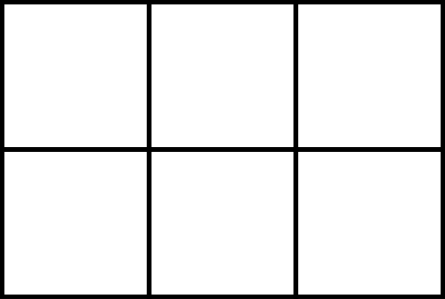First create a grid of the same size as the digits of the sum ie 265 x 36 will need a 3 by 2 grid.Note that the larger of the two numbers goes horizontally.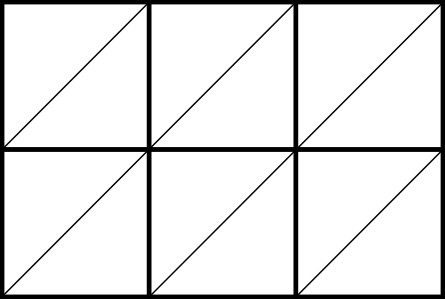Then add diagonal lines, going down to the left, to cut each square into two segments.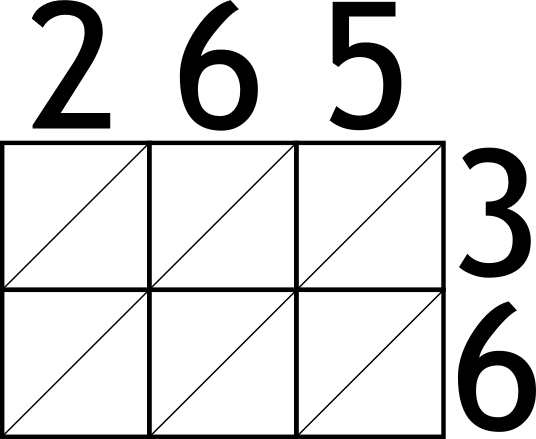Now write the longer number across the top of the grid and the smaller vertically downwards to the right of the grid, both with one digit per box.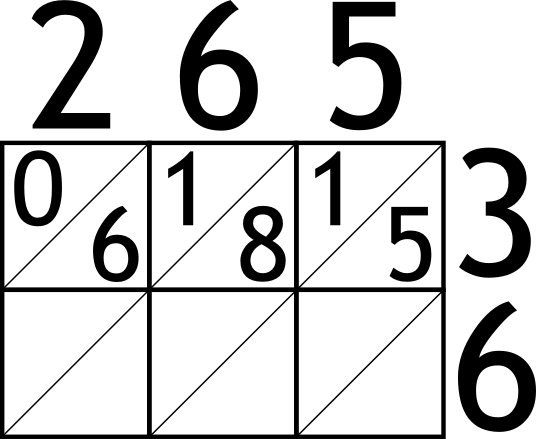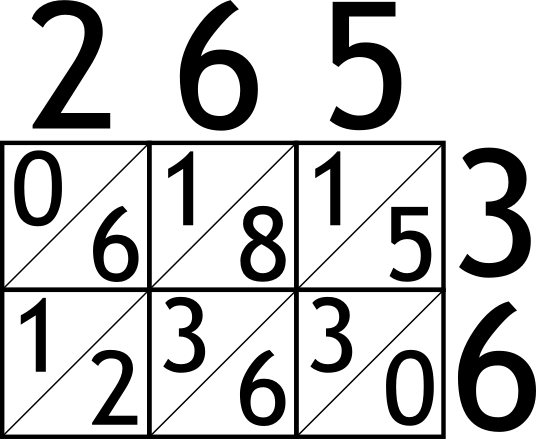Next multiply each pair of numbers, writing the answer in the corresponding box, one digit per segment.Note that a single digit value needs a zero as welleg 2 x 3 = 06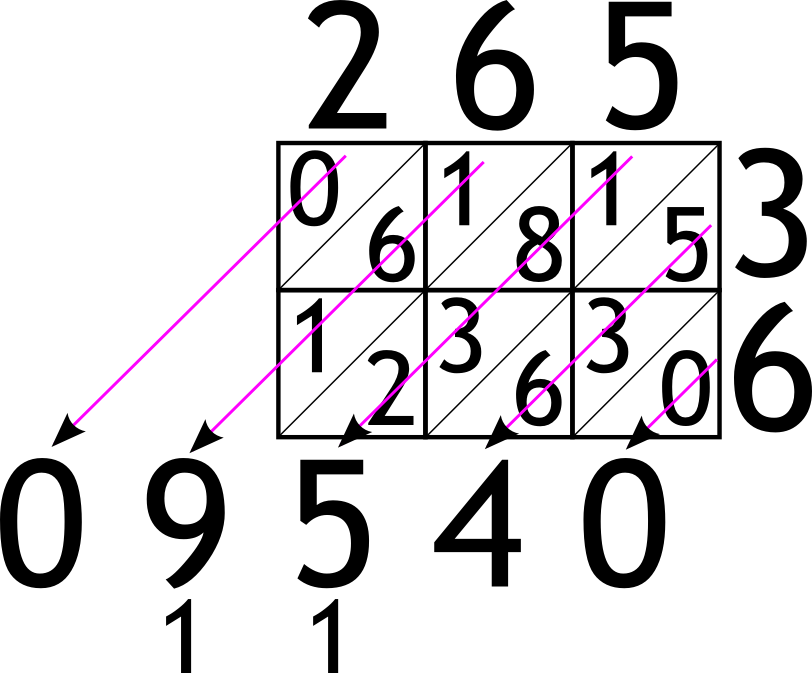Finally, add up down the diagonals, using carries where necessary, and that's the answer!